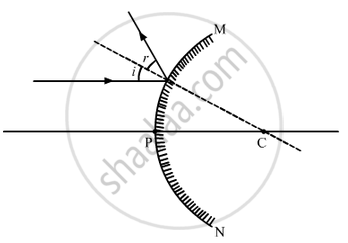# Draw a ray diagram to show the path of the reflected ray corresponding to an incident ray of light parallel to the principal axis of a concave mirror. Mark the angle of incidence and angle of reflection on it - Science

Draw a ray diagram to show the path of the reflected ray corresponding to an incident ray of light parallel to the principal axis of a concave mirror. Mark the angle of incidence and angle of reflection on it.

#### Solution

Ray diagram to show the path of the reflected ray corresponding to an incident ray of light parallel to the principal axis of a convex mirror:Concept: Concave Mirror
Is there an error in this question or solution?